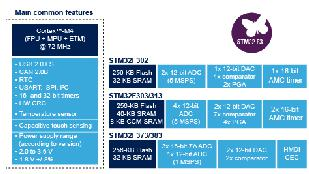STM32F37 Standard Peripheral bibliotheek  1.0 ST Microelectronics bibliotheek documentatie voor de STM32F37 Standard Peripheral Library
Output_Maximum_frequency_enumeration

## Macros

#define IS_GPIO_SPEED(SPEED)

## Enumerations

enum  GPIOSpeed_TypeDef { GPIO_Speed_Level_1 = 0x01, GPIO_Speed_Level_2 = 0x02, GPIO_Speed_Level_3 = 0x03 }

## Macro Definition Documentation

 #define IS_GPIO_SPEED ( SPEED )
Value:
(((SPEED) == GPIO_Speed_Level_1) || ((SPEED) == GPIO_Speed_Level_2) || \
((SPEED) == GPIO_Speed_Level_3))

## Enumeration Type Documentation

 enum GPIOSpeed_TypeDef
Enumerator
GPIO_Speed_Level_1

Medium Speed

GPIO_Speed_Level_2

Fast Speed

GPIO_Speed_Level_3

High Speed